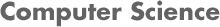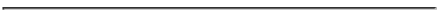### CS4341 Introduction to Artificial Intelligence Homework 2 and Sample Exam Problems A Term 2017

#### Prof. Carolina Ruiz and Ahmedul Kabir

Due Date: Canvas submission by Monday, Sept. 25th, 2017 at 11 am.This homework assignment consists of 2 parts:
1. Assigned problems from the textbook: You need to submit your solutions on Canvas.
2. Sample exam problems: No need to submit your solutions but you should study them for Exam 2. Try to solve these problems first as this will help you with the HW problems too!

1. #### Assigned HW problems from the textbook:

• Read Chapters/Sections 6, 7.1-7.5, 8, 9.3-9.4 and 10 of the textbook.

• Problems: Submit your answers on Canvas to the textbook problems listed below as "Required for the HW". Note that we have also listed other textbook problems that are required for the HW, but which would be good for you to try to solve in preparation for the exam (you don't need to submit solutions to these latter problems in your HW submission).

• Chapter 6: (pp. 230-233)

• Required for the HW:
• 6.2
• 6.4a
• 6.9
• 6.11
• 6.16

• Not required for the HW but useful to study for the exam:
• 6.3
• 6.6
• 6.15

• Chapter 7: (pp. 279-284)

• Required for the HW:
• 7.1
• 7.4: parts a, b, c, i, j. [All the parts of this problem are good exercises; we are picking some just to keep the hw shorter.]
• 7.10
• 7.12. (Note that you need to covert those sentences to clausal form first.)
• 7.17: part a

• Not required for the HW but useful to study for the exam:
• 7.5 (remember to prove both directions of the "if and only if")
• 7.6
• 7.7 (Note that the models must assign truth values each propositional letter A, B, C, and D, not just to the propositional letters appearing in the sentence. Also, note that A . B . C is shortcut for (A . B) . C, which is also equivalent to A . (B . C) )
• 7.9
• Determine whether the following sentence is valid, unsatisfiable, or neither. "!" means "not. Justify your answer with either truth tables, equivalent rewrites of the sentence, or both.
` (!! q => !p) => (p => !q) `

• Chapter 8: (pp. 315-321)

• Required for the HW:
• 8.10
• 8.11
• 8.23

• Not required for the HW but useful to study for the exam:
• 8.20

• Chapter 9: (pp. 360-365)

• Required for the HW:
• 9.9
• 9.6 and 9.13a

• Not required for the HW but useful to study for the exam:
• 9.10

• Chapter 10: (pp. 396-397)

• Required for the HW:
• 10.2
• 10.3

• Not required for the HW but useful to study for the exam:
• 10.4

2. #### Additional sample exam problems:

Practice for the exam by solving the previous exams and homework problems from Prof. Ruiz's offerings of this course listed below. Solve the problems on your own before looking at the provided solutions. No need to submit your answers as part of your homework submission.

Some of the problems below use another textbook's notation in which a letter precedeed by "?" means that the letter is a variable (and not a constant)). For example, "?a" is a variable.

• From Graduate AI Course Spring 2013:
• Homework 4 (with solutions): Problems 1 and 2.
• Homework 5 (with solutions): Problem 1.

• From B Term 2003:
• Exam 1 (with solutions): Problems IV and V.
• Project/HW 3 (with solutions): Part I.

• From C Term 2002:
• Exam 1 (with solutions): Problems II and III.
• Project 2 (with solutions): Part I.

• From D Term 2001:
• Exam 1 (with solutions): Problems IV and V.
• Exam 2 (with solutions): Problems 3 and 4.

• From C Term 2000:
• Exam 1 (with solutions): Problem III
• Exam 2 (with solutions): Problems I and II
• Homework 3 (with solutions): All problems
• Homework 4 (with solutions): Part 1: Problems 1, 2, and 3

• From A Term 1997: